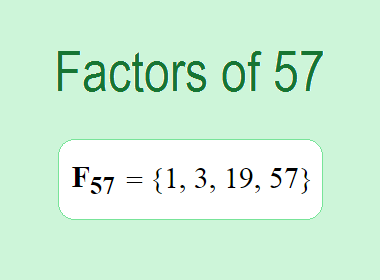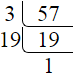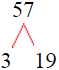# Factors of 57The factors of 57 are 1, 3, 19, and 57 i.e. F57 = {1, 3, 19, 57}. The factors of 57 are those numbers that can divide 144 without leaving a remainder.

To find the factors of 57, we can start by dividing 57 by the smallest possible factor, which is 1. If we divide 57 by 1, the result is 57. Therefore, 1 is a factor of 57. The next possible factor is 2. If we divide 57 by 2, we get a remainder of 1. Therefore, 2 is not a factor of 57.

The next possible factor is 3. If we divide 57 by 3, we get a remainder of 0. Therefore, 3 is a factor of 57. We can also check if 19 is a factor of 57. If we divide 57 by 19, we get a remainder of 0. Therefore, 19 is also a factor of 57.

Next, we can check if 4 is a factor of 57. If we divide 57 by 4, we get a remainder of 1. Therefore, 4 is not a factor of 57. We can continue this process for all the possible factors of 57.

Through this process, we can find that the factors of 57 are 1, 3, 19, and 57. These are the only numbers that can divide 57 without leaving a remainder.********************

********************

## Properties of the Factors of 57

The factors of 57 have some interesting properties. One of the properties is that the sum of the factors of 57 is equal to 80. We can see this by adding all the factors of 57 together:

1 + 3 + 19 + 57 = 80

Another property of the factors of 57 is that they are all odd numbers. This is because 57 is an odd number, and any even number cannot divide an odd number without leaving a remainder.

## Applications of the Factors of 57

The factors of 57 have several applications in mathematics. One of the applications is in finding the highest common factor (HCF) of two or more numbers. The HCF is the largest factor that two or more numbers have in common. For example, to find the HCF of 57 and 76, we need to find the factors of both numbers and identify the largest factor they have in common. The factors of 57 are 1, 3, 19, and 57. The factors of 76 are 1, 2, 4, 19, 38, and 76. The largest factor that they have in common is 19. Therefore, the HCF of 57 and 76 is 19.

Another application of the factors of 57 is in prime factorization. Prime factorization is the process of expressing a number as the product of its prime factors. The prime factors of 57 are 3 and 19, since these are the only prime numbers that can divide 57 without leaving a remainder. Therefore, we can express 57 as:

57 = 3 x 19

We can do prime factorization by division and factor tree method also. Here is the prime factorization of 57 by division method,57 = 3 x 19

Here is the prime factorization of 57 by factor tree method,57 = 3 x 19

## Conclusion

The factors of 57 are the numbers that can divide 57 without leaving a remainder. The factors of 57 are 1, 3, 19, and 57. The factors of 57 have some interesting properties, such as being odd numbers and having a sum of 80. The factors of 57 have several applications in mathematics, such as finding the greatest common factor and prime factorization.